Next: Context-free grammars Up: Grammars and Parsing Previous: The notion of a grammar

## Chomsky classification

The grammars of Example 3 have the nice following property: every production has the form A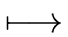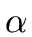where A is a non-terminal symbol andis a string of grammar symbols. These grammars are called context-free grammars and will be studied in the next section. They are one of the classes of the classification of Chomsky that we present now.

TYPE 1. The grammar G = (VT, VN, S, P) is of type 1 if every production has the form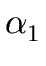A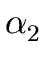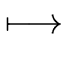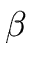where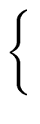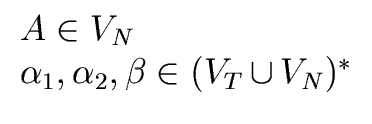(7)

Such a grammar is also called a context-sensitive grammar.

TYPE 2. The grammar G = (VT, VN, S, P) is of type 2 if every production has the form

 A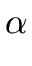where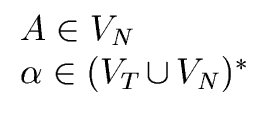(8)

Such a grammar is also called a context-free grammar.

TYPE 3. The grammar G = (VT, VN, S, P) is said right regular if every production has the form

 AaB  or  Aa    where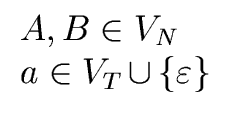(9)

The grammar G = (VT, VN, S, P) is said left regular if every production has the form

 ABa  or  Aa    where(10)

The grammar G is said regular or of type 3 if it is either right regular or left regular.

TYPE 0. The grammar G = (VT, VN, S, P) is of type 0 if it is not of type 1, 2 or 3.

THE TYPE OF A LANGUAGE. Let n be an integer in the range 0, 1, 2, 3. A language L over an alphabet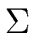is said of type n if it is generated by a grammar of type n and cannot be generated by a grammar of type n + 1. A language of type 2 is also said context-free (or algebraic) and a language of type 3 is also said regular.

REGULAR LANGUAGES. Let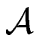= (, S, s0, F,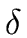) be a DFA. We define the grammar G = (, S, s0, P) such that

• its terminals are the letters of,
• its non-terminals are the states of the automaton,
• its start-symbol is the initial state of,
• its productions are defined as follows: for each x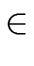and each sS such that(s, x) is defined we set the rule sxs' where s' =(s, x). Moreover if s' is a final state we set the rule s'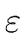.
It is not hard to prove that the language recognized byis the language generated by G. Observe that G is a regular grammar. Conversly, one can build a FA from a regular grammar such that their associated languages match. Hence we can state the following theorem.

Theorem 1   A grammar G is regular iff the language generated by G is recognized by finite automata.

Example 4   Consider= {a, b} and the language L overdenoted by the regular expression r = (a + b) * abb. From r one can easily build the NFA shown on Figure 4. Then applying the subset algorithm one obtains the DFA shown on the same picture.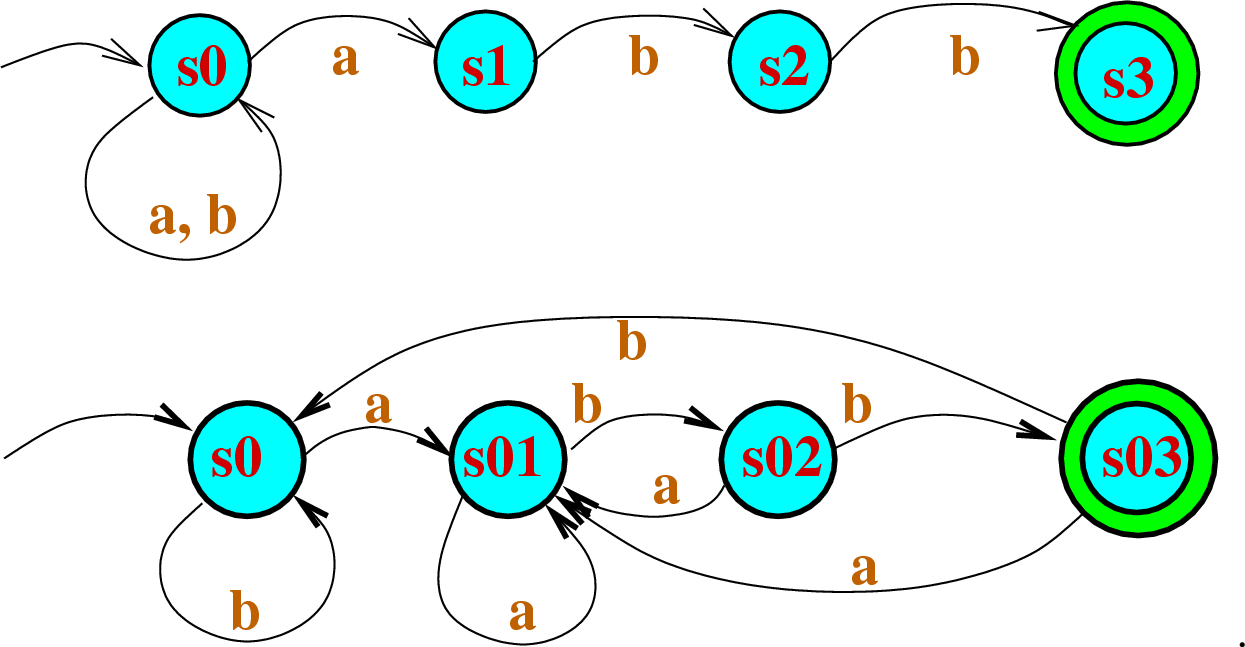The leads us to the following grammar:

 s0as01  |  bs0 s01as01  |  bs02 s02as01  |  bs03 s03as01  |  bs0  |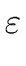(11)

CONTEXT-FREE LANGUAGES. We start with two examples of context-free languages that we met already. Then we give a pumping-lemma for context-free languages as Theorem 2.

Example 5   Consider= {(,)} and let L be the language of the well parenthesized expressions over. So these expressions are only made of parentheses and satisfy Condition 2 of Remark 1. We consider the grammar G = (,{S}, S, P) where the set of productions P is given by

 S(S)S  |(12)

Let L' be the language generated by G. Let us show that L is L'. By induction (on the length of a generated word) it is easy to prove that every word in L' is a well parenthesized expression, so L'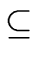L. Conversly by induction on the length of a well parenthesized expression, it is easy to prove that all such expressions can be generated by G, so LL'. Hence L = L'.

Example 6   Consider= {a, b} and L = {anbn  |  n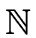}. The language L is of type 2. Indeed we already know that L is not recognized by FA and thus it is not of type 3. It is easy to show that L can be generated by the following context-free grammar.
 SaSb |Theorem 2 (Bar-Hillel, Perles, Shamir)   Let G = (VT, VN, S, P) be a context-free grammar. There exists an integer N such that for every word m generated by G with length | m |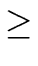N there exist words u, v, w, x, y over VT such that

 m  =  u v w x y    with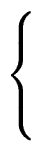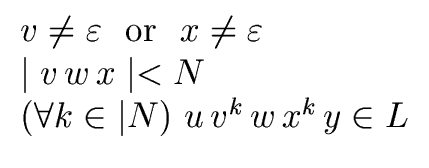(13)

Example 7   Consider the alphabet= {a, b, c} = VT and the language

 L  =   {anbncn  |  n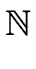} (14)

over. We define VN = {S, T, U, W, X, Y, Z, B}. The grammar G = (VT, VN, S, P) with P given by

 Sabc SaTBc TBUB UBUW UWBW BWBT TcXBcc BXBY ZYZB ZBXB aXaaT aXaa Bb BYZY
(15)

generates the language L. Theorem 2 can be used to prove that L is not a context-free language. Therefore L is a language of type 1.Next: Context-free grammars Up: Grammars and Parsing Previous: The notion of a grammar
Marc Moreno Maza
2004-12-02